Get instant live expert help with Excel or Google Sheets“My Excelchat expert helped me in less than 20 minutes, saving me what would have been 5 hours of work!”

#### Post your problem and you’ll get expert help in seconds.

Your message must be at least 40 characters
Our professional experts are available now. Your privacy is guaranteed.

# How to use the Excel ISNUMBER and ISNUMERIC VBA Function

We can use the Excel ISNUMBER and ISNUMERIC VBA functions to detect numeric values in Excel and VBA programming. Both functions return TRUE if the cell contains a number and FALSE, if not. However, the Excel ISNUMERIC function is a VBA function whereas the ISNUMBER function is a worksheet function. Both functions can also yield different results in similar circumstances.

In this tutorial, we will learn how to use the EXCEL ISNUMBER and the ISNUMERIC VBA functions.

## Syntax for EXCEL ISNUMBER Function

`=ISNUMBER(value)`

Where value is the specified cell, formula, function or value to test. The ISNUMBER function checks if a value is stored as a number.

## Syntax for EXCEL ISNUMERIC function

`IsNumeric(expression)`

Where expression is evaluated as a number. The Excel VBA ISNUMERIC checks if a value can be converted to a number.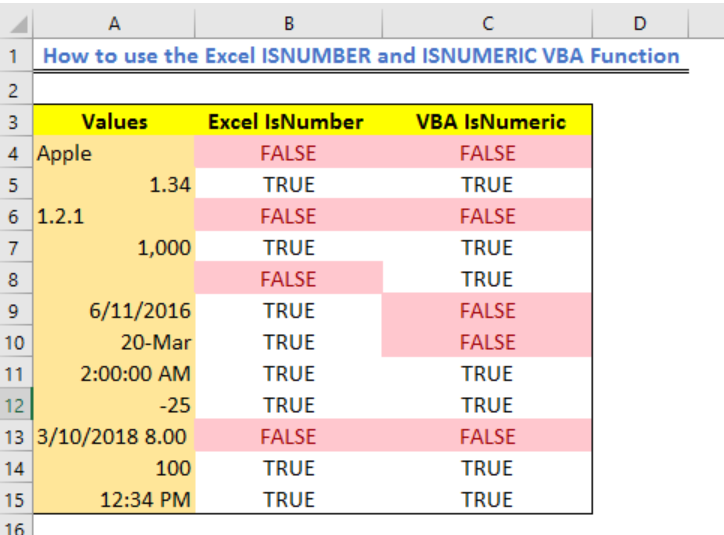Figure 1 – Result of excel check if number is True or False

## Setting up Data and VBA for ISNUMERIC and Excel ISNUMBER functions

• We will set a data table as shown below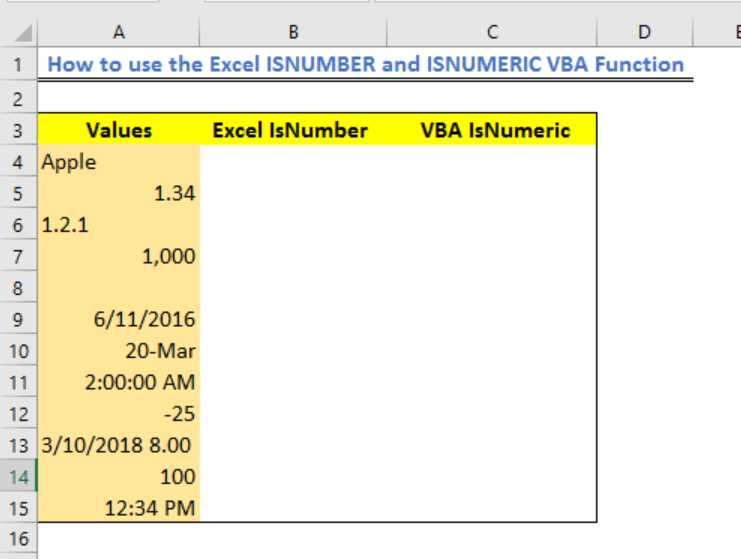Figure 2 –  Setting Data for Excel ISNUMBER and ISNUMERIC function

• We will click on Developer and select Visual Basic

• Next, we will click on Insert and select Module

• In the new VBA window, we will enter this Macro code below

`Function IsNumericTest(TestCell As Variant)`

`'Use VBA to test if a cell is numeric via a function`

`If IsNumeric(TestCell) Then 'if TestCell is True`

`    IsNumericTest = True 'Cell is a number`

`Else`

`    IsNumericTest = False 'Cell is not a number`

`End If`

`End Function`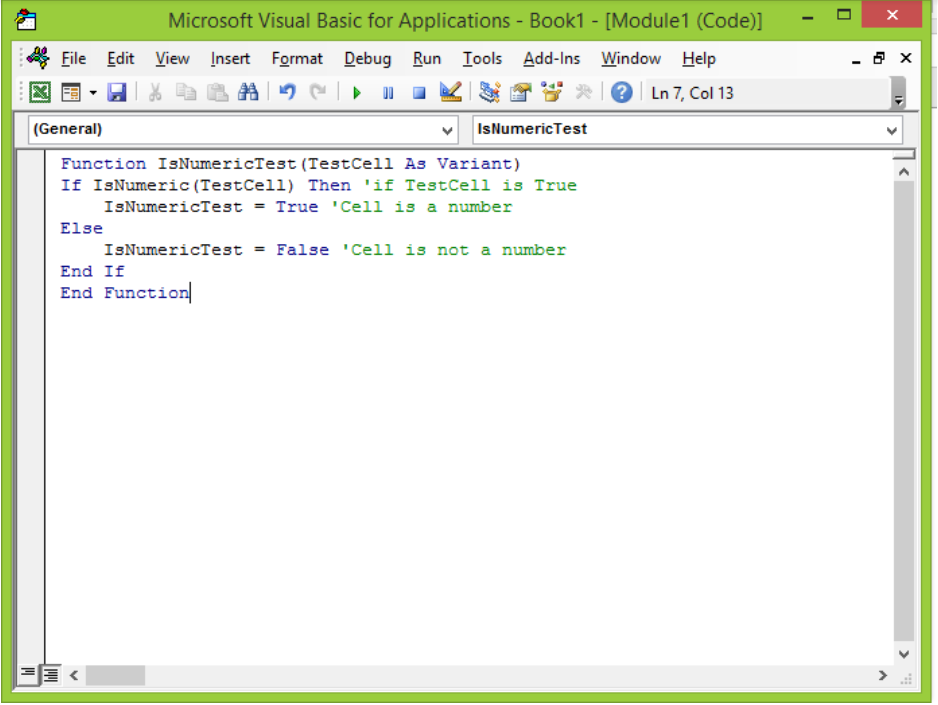Figure 3 – Excel ISNUMERIC VBA

## Testing Data using Excel VBA ISNUMERIC and ISNUMBER functions

We will test Column A with the Excel ISNUMBER function in Column B and Excel VBA IsNumeric() function in Column C.

1. To check with the ISNUMBER function;

• In Cell B4, we will enter the formula below and press the Enter key

`=ISNUMBER(A4)`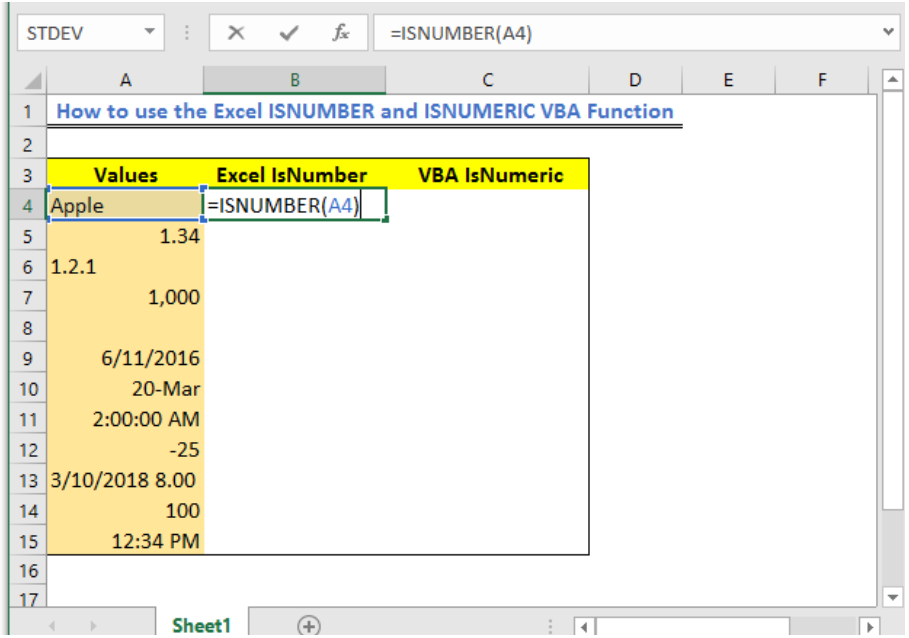Figure 4 – Excel ISNUMBER

• We will have this result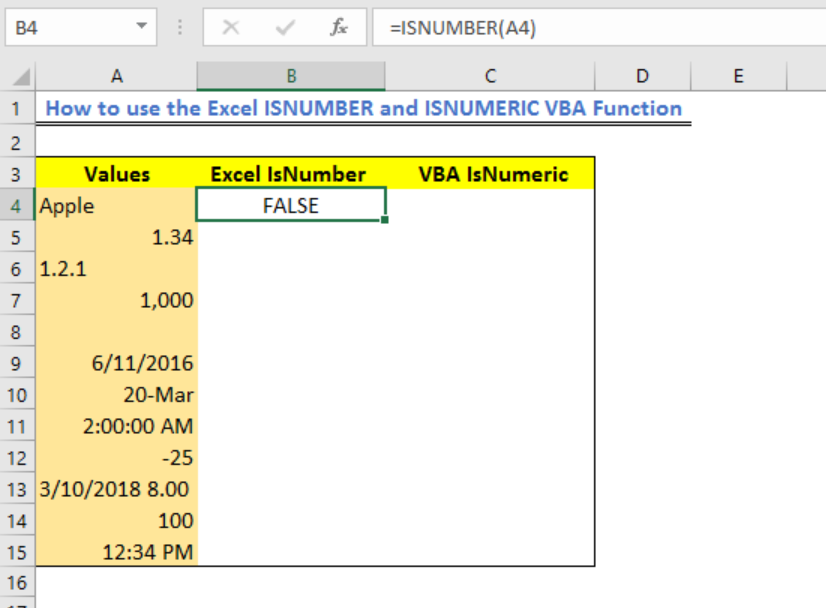Figure 5 – Using the Excel ISNUMBER function

• We will click again on Cell B4 and using the fill handle tool, we will drag the formula down the column to get this result: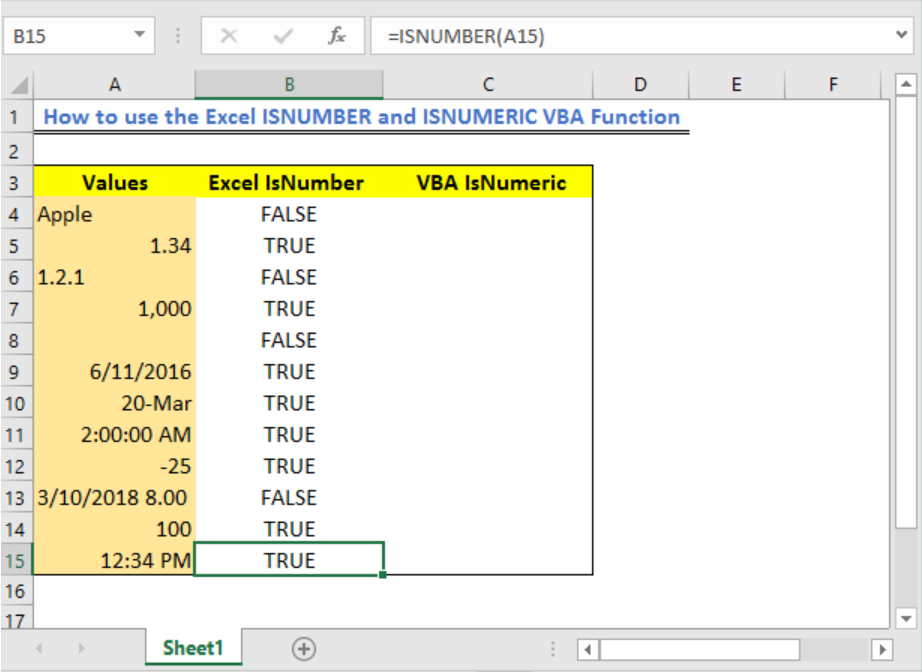Figure 6 – ISNUMBER function in Excel

2. For the IsNumeric function test

• We will click on Cell C4, enter the formula below and press the enter key

`=IsNumericTest(A4)`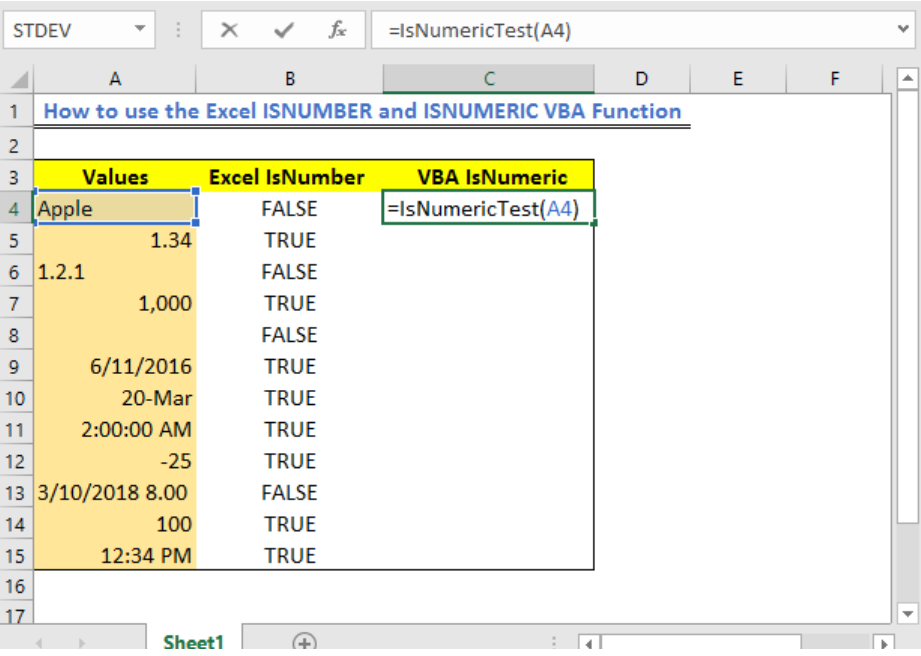Figure 7 – ISNUMERIC VBA

• We will have this result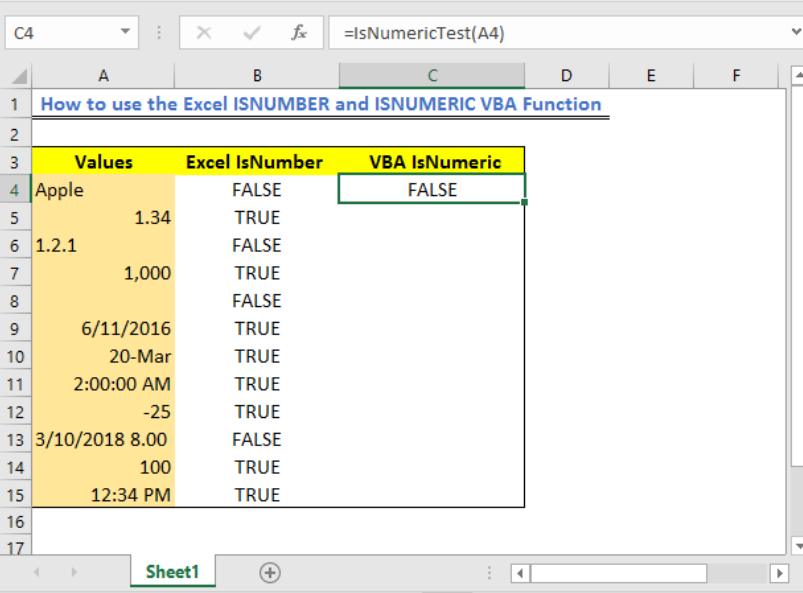Figure 8 – VBA ISNUMERIC

• Now, we will click on Cell C4 and using the fill handle tool, we will drag the formula down the column to get this result: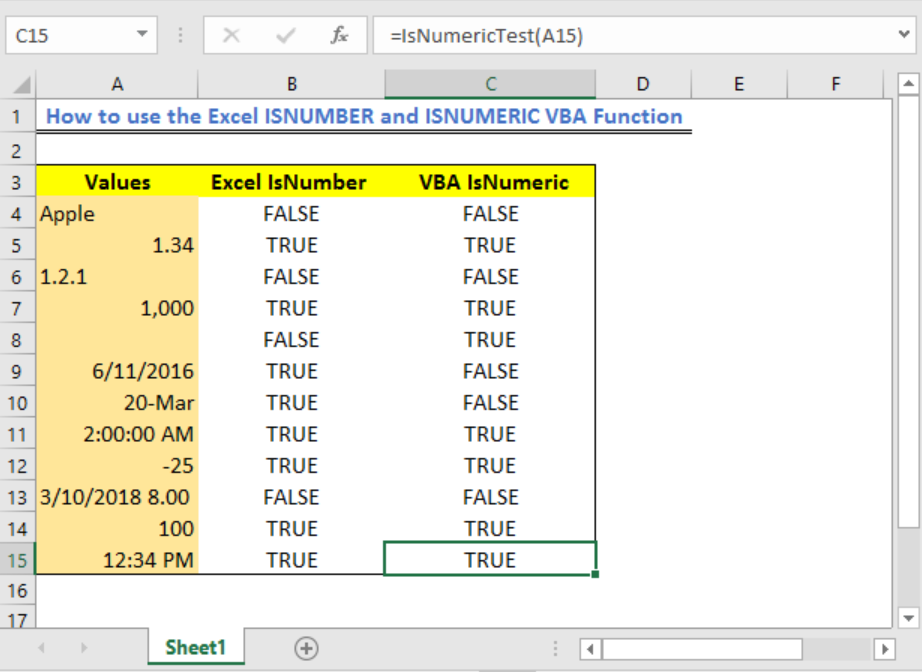Figure 9 – Excel VBA ISNUMERIC

## Explanation

In our example, this is the different results given by the two functions.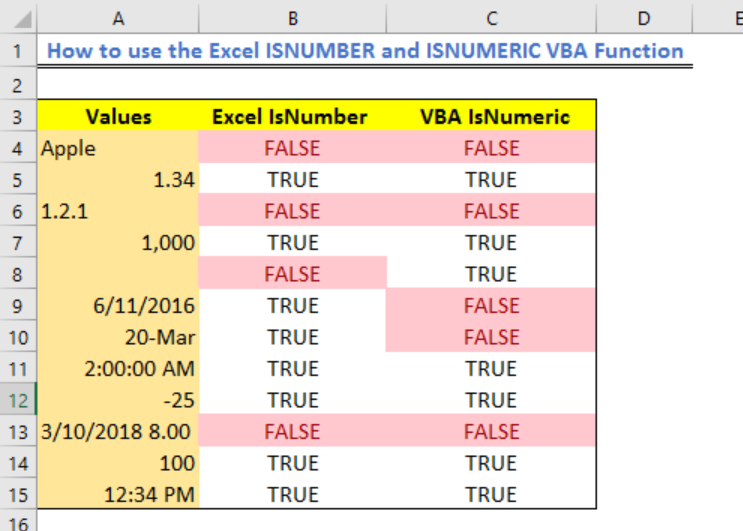Figure 10 – Using Conditional formatting for ISNUMBER versus ISNUMERIC in Excel

By Comparing results using both functions, we will find that

• The Excel IsNumeric function considers empty numeric cells but the Excel ISNUMBER function does not
• The Excel ISNUMBER function finds dates entered with texts and characters as numbers because it is stored as numbers whereas the Excel IsNumeric does not

## Instant Connection to an Expert through our Excelchat Service

Most of the time, the problem you will need to solve will be more complex than a simple application of a formula or function. If you want to save hours of research and frustration, try our live Excelchat service! Our Excel Experts are available 24/7 to answer any Excel question you may have. We guarantee a connection within 30 seconds and a customized solution within 20 minutes.